# Generic small area estimation

#### 2022-07-07

In this vignette, we illustrate the use of the smoothArea and smoothUnit functions for generic small area estimation of outcomes other than under-five mortality rates.

First, we load the necessary packages and the necessary data. The required package INLA is not in a standard repository, so we install if it is not available. The survey package will be used to generate direct estimates, while dplyr and tidyr will be used to facilitate data manipulation.

library(sae)
library(SUMMER)
library(survey)
library(dplyr)
library(tidyr)
if (!isTRUE(requireNamespace("INLA", quietly = TRUE))) {
install.packages("INLA", repos=c(getOption("repos"),
}

# Area level models

### Income data

First, we compare our results with those obtained using the sae package using examples presented in the sae vignette. Molina and Marhuenda (2015) generate an artificial dataset on income and other related variables to illustrate the use of area level models in the sae package. In their simulated example, the objective is to estimate prevalence of poverty in Spanish counties.

data("incomedata")
data("sizeprov")
data("sizeprovedu")
povertyline <- 0.6 * median(incomedata$income) # 6557.143 incomedata$poor <- as.integer(incomedata$income < povertyline) The incomedata data frame contains information on 17199 observations of individuals in 52 Spanish provinces. Income values and sampling weights are provided for each individual along with covariate information including age group and education level. Molina and Marhuenda (2015) define the poverty line z, and calculate an indicator variable with value 1 if the corresponding income value is below the poverty line and 0 otherwise. #### Direct estimation with sae The sae::direct function computes the Horvitz-Thompson estimator of domain means, given by, $\widehat{\overline{Y}}_{d}^{\text{DIR}}=\frac{1}{N_d}\sum_{k\in S_d}w_{k}y_{k}$ where $$N_d$$ is the population size of domain $$d$$, $$S_d$$ is the set of sampled observations in domain $$d$$, $$w_{k}$$ is the sampling weight for unit $$k$$, and $$y_{k}$$ is the observation for unit $$k$$, for all $$k \in S_d$$. The sae::direct function also estimates standard deviation and coefficient of variation for each domain. Popn <- sizeprov[, c("provlab", "Nd")] sae.DIR <- sae::direct(y = incomedata$poor, dom = incomedata$provlab, sweight = incomedata$weight, domsize = Popn)
knitr::kable(head(sae.DIR), digits = 3)
Domain SampSize Direct SD CV
Alava 96 0.255 0.048 19
Albacete 173 0.141 0.030 22
Alicante 539 0.205 0.022 11
Almeria 198 0.265 0.041 15
Avila 58 0.055 0.026 46

#### Direct estimation with survey

We can similarly use the survey::svyby function to compute the Horvitz-Thompson estimates:

incomedata$pop <- sum(sizeprov$Nd[match(incomedata$provlab, sizeprov$provlab)])
design <- survey::svydesign(ids = ~1, weights = ~weight, data = incomedata,
fpc = ~pop)

# estimate area totals
svy.DIR <- survey::svyby(~poor, ~provlab, design, svytotal)

# calculate corresponding area mean estimates
svy.DIR$prov_pop <- sizeprov$Nd[match(svy.DIR$provlab, sizeprov$provlab)]
svy.DIR$poor_mean <- svy.DIR$poor/svy.DIR$prov_pop svy.DIR$poor_mean_se <- svy.DIR$se/svy.DIR$prov_pop
select(provlab, poor_mean, poor_mean_se), digits = 3)
provlab poor_mean poor_mean_se
Alava Alava 0.255 0.048
Albacete Albacete 0.141 0.030
Alicante Alicante 0.205 0.022
Almeria Almeria 0.265 0.041
Avila Avila 0.055 0.026

#### Fay-Herriot model

The Fay-Herriot model is a basic area level model used to obtain small area estimators Fay and Herriot (1979). The Fay-Herriot model is often specified as a two-stage model; the first stage is the sampling model and the second stage is the linking model.

First stage:

Let $$\widehat\theta^{\text{DIR}}_d$$ be a direct estimator of $$\theta_d$$: $\widehat\theta^{\text{DIR}}_d=\theta_d+\epsilon_d;\hspace{2em}\epsilon_d\sim_{ind}N(0,V_d),\hspace{2em}d=1,\ldots, D,$

where $$V_d$$ is the known sampling variance of the direct estimator $$\widehat{\theta}^{\text{DIR}}_d$$.

Second stage:

$\theta_d = \mathbf{x}_d^T\boldsymbol\beta+u_d,\hspace{2em}u_d\sim_{ind}N(0,\sigma_u^2)\hspace{2em}d=1,\ldots, D,$

where $$\sigma_u^2$$ (between-area residual variance) is estimated. In this basic Fay-Herriot model, the area-specific random effects $$u_d$$ are assumed to be independent between areas.

#### Implementing Fay-Herriot model with sae

Below, we provide a quantile-quantile plot comparing the direct estimates to a Gaussian distribution. Here the observed quantiles align well with those from a Gaussian distribution, which lends some support to the basic IID model.

par(pty = "s")
muD <- mean(sae.DIR$Direct) sdD <- sd(sae.DIR$Direct)
qqnorm((sae.DIR$Direct - muD)/sdD, main = "") abline(0, 1, col = "red")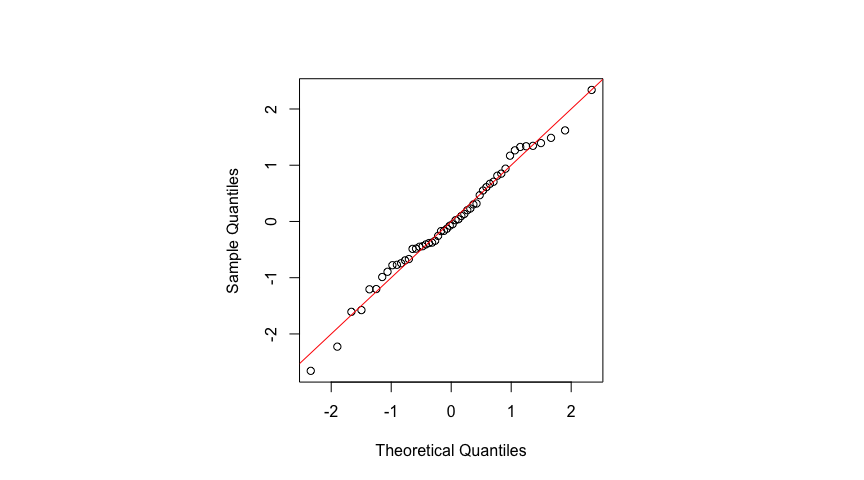The sae::mseFH function calculates the empirical best linear unbiased predictors (EBLUP) for all domain means as well as their estimated MSEs. sae.FH <- sae::mseFH(sae.DIR$Direct ~ 1, sae.DIR$SD^2) #### Implementing Fay-Herriot model in SUMMER The smoothArea function compute direct estimates and then produces smoothed estimates using a Bayesian Fay-Herriot model. The main arguments of interest are: • formula: describing the response variable and any area-level covariates • domain a one-sided formula with the variable containing domain labels on the right. The domain labels variable should be contained in the dataset used to generate the design. • design: a survey.design object containing survey data and specifying the survey design. In addition, other commonly used optional arguments include: • Amat: Optional adjacency matrix if a spatial smoothing model is desired. • responseType: either "gaussian" or "binary" depending on the type of response variable. If "binary" is specified, a logit transform will be applied to the direct estimates before smoothing. • direct.est: If desired, the direct estimates may be specified and smoothing will be applied directly to the direct estimates. • X.area: Areal covariates data frame. • domain.size: Domain size data frame used for computing direct estimates if domain sizes are known. Other optional arguments can be specified to change the priors and are described further in the documentation. We obtain direct estimates and smoothed estimates below, fitting the Fay-Herriot model to the untransformed direct estimates. # fit the model domain.size <- sizeprov[, c("provlab", "Nd")] colnames(domain.size) <- "size" summer.FH <- smoothArea(formula = poor ~ 1, domain = ~provlab, design = design, domain.size = domain.size) The fitted parameters from sae (obtained via likelihood-based methods) and estimated parameter posterior distribution from SUMMER (obtained from Bayesian methods, implemented via INLA) are in reasonable agreement. The estimated intercept $$\beta_0$$ from sae is 0.2 ; the posterior median of $$\beta_0$$ from SUMMER is 0.2. In the absence of strong priors, fixed effects are usually in close agreement, with the posterior being symmetric. The estimated precision $$1/\sigma_u^2$$ from sae is 281.35 , while the posterior median of $$1/\sigma_u^2$$ from SUMMER is 273.4. The differences are larger here, but the posterior for the variance is skewed, and we would expect the posterior median to be smaller than the REML estimate. The area estimates and measures of uncertainty are in close agreement, however. We first illustrate the shrinkage of the EBLUP estimates, and the reduced uncertainty: par(mfrow = c(1, 2)) plot(sae.DIR$Direct, sae.FH$est$eblup,
xlab = "Direct estimates",ylab = "sae package",
xlim=c(min(sae.DIR$Direct, sae.FH$est$eblup),max(sae.DIR$Direct, sae.FH$est$eblup)),
ylim=c(min(sae.DIR$Direct, sae.FH$est$eblup),max(sae.DIR$Direct, sae.FH$est$eblup)),
main = "Small area estimates")
abline(0,1,col="red")
plot(sae.DIR$SD^2, sae.FH$mse,
xlab = "Direct estimates",ylab = "sae package",
xlim=c(min(sae.DIR$SD^2, sae.FH$mse),max(sae.DIR$SD^2, sae.FH$mse)),
ylim=c(min(sae.DIR$SD^2, sae.FH$mse),max(sae.DIR$SD^2, sae.FH$mse)),
main = "Estimates of uncertainty")
abline(0,1,col="red")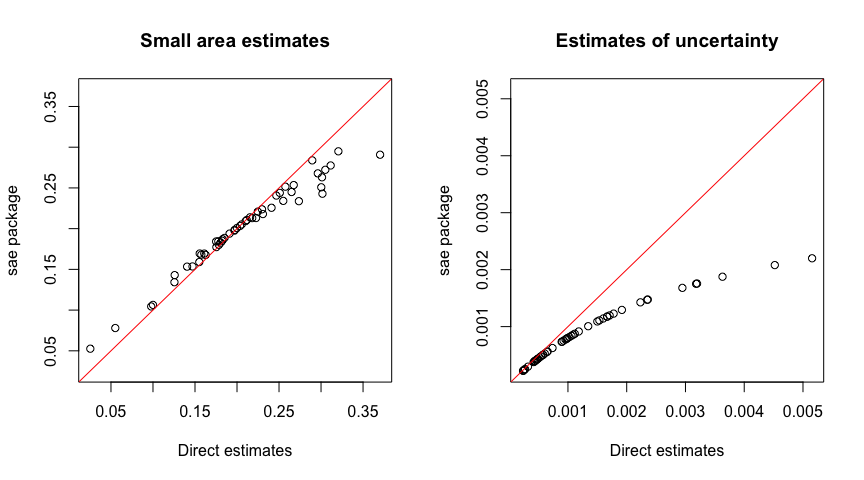Now compare EBLUP and HB, using posterior variance from SUMMER and estimated MSE from sae to measure uncertainty:

par(mfrow = c(1, 2))
plot(sae.FH$est$eblup, summer.FH$s.dir.iid.est$median,
xlab = "SAE package",ylab = "SUMMER package",
main = "Small area estimates")
abline(0,1,col="red")
plot(sae.FH$mse, summer.FH$s.dir.iid.est$var, xlab = "sae package mse", ylab = "SUMMER package model variance", main = "Estimates of mse/variance") abline(0,1,col="red")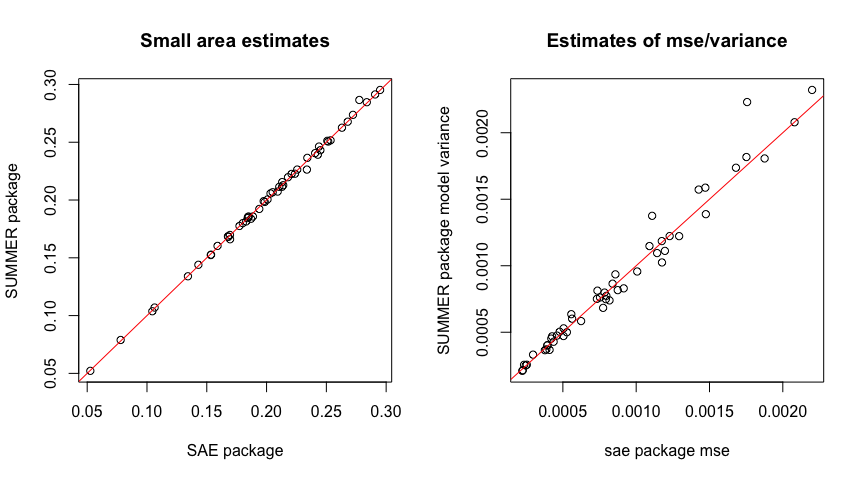### Milk expenditures Molina and Marhuenda (2015) also use a real dataset on milk expenditures Arora and Lahiri (1997) to illustrate the implementation of area level models in the sae package. The milk dataset contains information on direct estimators for area level expenditures on milk based on data from the dairy survey component of the Consumer Expenditure Survey conducted by the U.S. Census Bureau. Weights are based on inverse sampling probabilities and adjusted for non-response/post-stratification. data(milk) milk$Var <- milk$SD^2 knitr::kable(head(milk)) SmallArea ni yi SD CV MajorArea Var 1 191 1.10 0.16 0.15 1 0.03 2 633 1.07 0.08 0.07 1 0.01 3 597 1.10 0.08 0.07 1 0.01 4 221 0.63 0.11 0.17 1 0.01 5 195 0.75 0.12 0.16 1 0.01 6 191 0.98 0.14 0.14 1 0.02 Here, SmallArea denotes areas of inferential interest, yi gives the direct estimates, ni the sample sizes, SD and CV give the estimated standard deviation and coefficient of variation, and MajorArea corresponds to major areas (US regions defined by You and Chapman (2006)). #### Results using sae attach(milk) sae.FH.milk <- mseFH(yi ~ as.factor(MajorArea), SD^2) #### Results using SUMMER Since the milk dataset only provides area level direct estimates and not unit level data, we use the direct.est argument as input. # Major Area fixed effects Xmat <- milk[, c("SmallArea", "MajorArea")] Xmat$MajorArea <- as.factor(Xmat$MajorArea) # format direct estimates for SUMMER milk.dir <- milk[, c("SmallArea", "yi", "Var")] # Fit the model with Major Area intercepts summer.FH.milk<- smoothArea(formula = yi~MajorArea, direct.est = milk.dir, X.area = Xmat, domain = ~SmallArea) Again note that the posterior median for $$\sigma_u^2$$ is larger than the REML estimate. # random effects precision 1 / sae.FH.milk$est$fit$refvar
##  54
summer.FH.milk$s.dir.iid.fit$summary.hyperpar[,c(4)]
##  54

# estimated fixed effects
sae.FH.milk$est$fit$estcoef ## beta std.error tvalue pvalue ## X(Intercept) 0.97 0.069 14.0 2.8e-44 ## Xas.factor(MajorArea)2 0.13 0.103 1.3 2.0e-01 ## Xas.factor(MajorArea)3 0.23 0.092 2.5 1.4e-02 ## Xas.factor(MajorArea)4 -0.24 0.082 -3.0 3.1e-03 summer.FH.milk$s.dir.iid.fit$summary.fixed[,c(1:5)] ## mean sd 0.025quant 0.5quant 0.97quant ## (Intercept) 0.97 0.071 0.830 0.97 1.102 ## MajorArea2 0.13 0.105 -0.072 0.13 0.333 ## MajorArea3 0.23 0.094 0.041 0.23 0.404 ## MajorArea4 -0.24 0.083 -0.405 -0.24 -0.084 Again, we observe good agreement. par(mfrow = c(1, 2)) plot(sae.FH.milk$est$eblup[as.numeric(summer.FH.milk$s.dir.iid.est$domain)], summer.FH.milk$s.dir.iid.est$median, xlab = "sae package", ylab = "SUMMER package", main = "Small area estimates") abline(0,1,color="red") plot(sae.FH.milk$mse[as.numeric(summer.FH.milk$s.dir.iid.est$domain)],
summer.FH.milk$s.dir.iid.est$var,
xlab = "sae package mse",
ylab = "SUMMER package model variance",
main = "Estimates of mse/variance")
abline(0,1,col="red")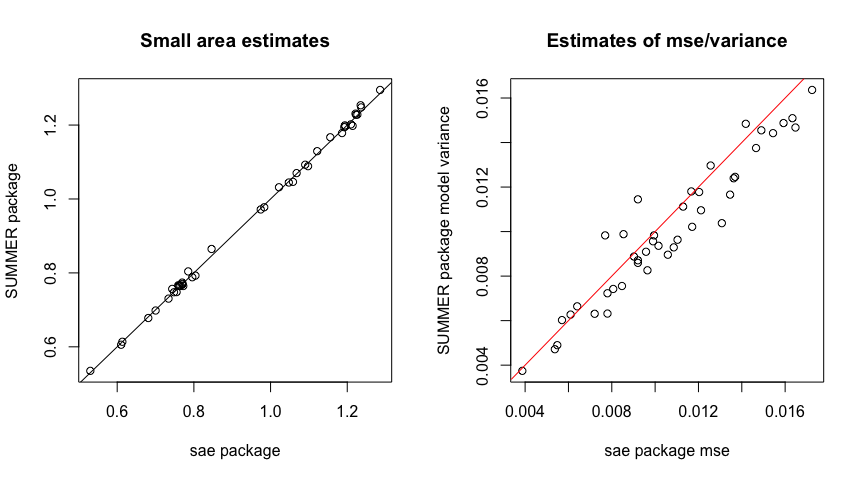### Spatial Fay-Herriot

The sae package also provides tools for implementing a spatial version of the Fay-Herriot model which assumes that the vector of area specific effects follows a first order simultaneous autoregressive, or SAR(1), process: $\mathbf{u}=\rho_1\mathbf{Wu}+\boldsymbol\epsilon,\hspace{1em}\boldsymbol\epsilon\sim N(\mathbf{0}_D,\sigma_I^2\mathbf{I}_D),$ where $$\mathbf{I}_D$$ is the identity matrix for the $$D$$ areas and $$\mathbf{0}_D$$ is a vector of zeroes of size $$D$$. Additionally, $$\rho_1\in(-1,1)$$ is an autoregression parameter and $$\mathbf{W}$$ is an adjacency matrix (with rows standardized to sum to 1).

The sae::mseSFH function estimates the unknown variance parameters, the resulting EBLUP small area estimators, and then uses bootstrap methods to estimate the MSE of the estimators.

To illustrate the use of this function, Molina and Marhuenda (2015) consider a synthetic dataset concerning grape production surface area for 274 Italian municipalities. Below we load the relevant objects from the sae package. The grapes dataset containes direct estimators of the mean surface area in hectares for grape production in each municipality (grapehect), the sampling variance of these direct estimators (var), and relevant covariates including number of working dats and overall agrarian surface area. The grapesprox object contains the relevant adjacency matrix representing the municipalities’ neighborhood structure.

data("grapes")
data("grapesprox")

#### Results using sae

sae.FH.grapes <- sae::mseSFH(grapehect ~ area + workdays - 1, var, grapesprox, data = grapes)

results <- data.frame(DIR = grapes$grapehect, eblup.SFH = sae.FH.grapes$est$eblup, mse = sae.FH.grapes$mse)
# reorder results for comparison later
results$area_name <- paste0('area_', rownames(results)) #### Results using SUMMER The smoothSurvey function also allows the use of a model with spatially correlated area effects, but the default implementation assumes a BYM2 model for $$\mathbf{u}$$ rather than a simultaneous autoregressive model as in the SFH model implemented in sae. # create area_name as SUMMER requires rownames of A_mat to match area variable grapes$area_name <- paste0('area_', rownames(grapes))
Amat_grapes <- as.matrix(grapesprox)
rownames(Amat_grapes) <- colnames(Amat_grapes) <- grapes$area_name X_grapes <- grapes[,c('area_name', 'area', 'workdays')] # format direct estimates for SUMMER grapes.dir <- grapes[, c(5, 1, 4)] # scale direct estimates for use with INLA grapes.dir$grapehect <- grapes.dir$grapehect / 10 grapes.dir$var <- grapes.dir$var/ 100 summer.FH.grapes<- smoothArea(formula = grapehect~area + workdays, direct.est = grapes.dir, X.area = X_grapes, domain = ~area_name, Amat = Amat_grapes) Despite the differing models, we again observe good agreement with the estimates, though less so with the estimates of uncertainty, which is interesting. par(mfrow = c(1, 2)) #??? plot(results$eblup.SFH,
summer.FH.grapes$s.dir.sp.est$median * 10,
xlab = "sae package",
ylab = "SUMMER package",
main = "Small area estimates")
abline(0,1, col = 'red')
plot(results$mse, summer.FH.grapes$s.dir.sp.estvar * 100, xlab = "sae package mse", ylab = "SUMMER package model variance", main = "Estimates of mse/variance") abline(0,1, col = 'red')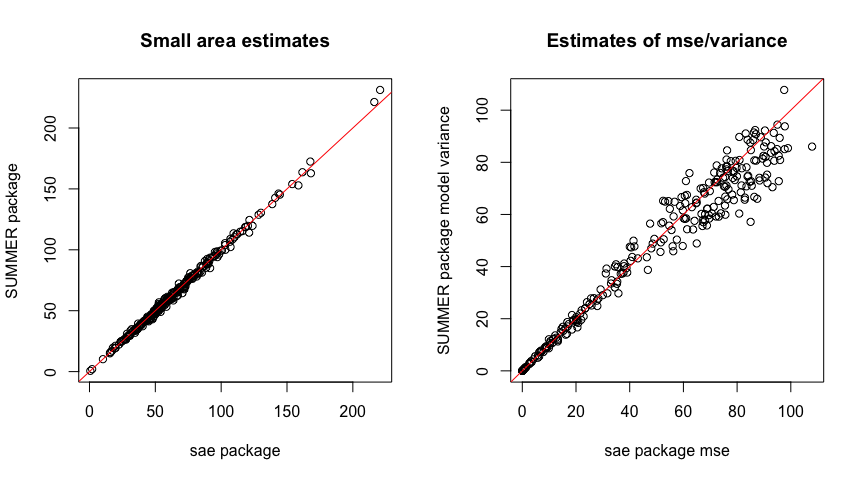# Unit Level Models The nested error model, introduced by Battese, Harter, and Fuller (1988), uses auxiliary data at the unit level. Nested error model: $y_{dk}=\mathbf{x}_{dk}^T\boldsymbol\beta+u_d+\epsilon_{dk},\hspace{1em}u_d\sim_{ind}N(0,\sigma_u^2),\hspace{1em}\epsilon_{dk}\sim_{ind}N(0,\sigma_\epsilon^2)$ Here $$u_d$$ are area random effects and $$\epsilon_{dk}$$ are unit level errors. This model assumes there is no sample selection bias. The sae package conducts estimation of domain means by first estimating variance parameters $$\sigma^2_u$$ and $$\sigma^2_\epsilon$$. Next, given known variance parameters, domain means $$\theta_d$$ are predicted by calculating the EBLUPs. The area fitted values are: $\widehat{y}_d^{\text{EBLUP}} = f_d \overline{y}_{dS} + (\overline{X}_d-f_d \overline{x}_{dS})\widehat{\beta} + (1-f_d)\widehat{u}_d,$ where • $$f_d=n_d/N_d$$ is the domain sampling fraction. • $$\overline{y}_{dS}$$ is the mean response in the sampled units. • $$\overline{x}_{dS}$$ is the mean of the covariates in the sampled units. • $$\overline{X}_d$$ is the mean of the covariates in the population. • $$\widehat{u}_d$$ is the estimated random effect. ## Corn and Soy Production The cornsoybean and cornsoybeanmeans datasets contain info on corn and soy beans production in 12 Iowa counties Battese, Harter, and Fuller (1988). The objective here is use satellite imagery of the number of pixels assigned to corn and soy to estimate the hectares grown of corn. • SampSegments: sample size. • PopnSegments: population size. • MeanCornPixPerSeg: county mean of the number of corn pixels (satellite imagery). • MeanSoyBeansPixPerSeg county mean of the number of soy beans (satellite imagery) pixels. The variables MeanCornPixPerSeg and MeanSoyBeansPixPerSeg provide the known county means of the auxiliary variables. We load the sample data: data("cornsoybean") head(cornsoybean,n=10) ## County CornHec SoyBeansHec CornPix SoyBeansPix ## 1 1 166 8.1 374 55 ## 2 2 96 106.0 209 218 ## 3 3 76 103.6 253 250 ## 4 4 185 6.5 432 96 ## 5 4 116 63.8 367 178 ## 6 5 162 43.5 361 137 ## 7 5 152 71.4 288 206 ## 8 5 162 42.5 369 165 ## 9 6 93 105.3 206 218 ## 10 6 150 76.5 316 221 Next, we load the population auxiliary information: data("cornsoybeanmeans") Xmean <- data.frame(cornsoybeanmeans[, c("CountyIndex", "MeanCornPixPerSeg", "MeanSoyBeansPixPerSeg")]) Popn <- data.frame(cornsoybeanmeans[, c("CountyIndex", "PopnSegments")]) head(Xmean) ## CountyIndex MeanCornPixPerSeg MeanSoyBeansPixPerSeg ## 1 1 295 190 ## 2 2 300 197 ## 3 3 290 205 ## 4 4 291 220 ## 5 5 318 188 ## 6 6 257 247 The sae::pbmseBHF function obtains EBLUPs under the nested error model and then uses a parametric bootstrap approach to estimate MSEs. cornsoybean <- cornsoybean[-33, ] # remove outlier sae.bhf <- pbmseBHF(CornHec ~ CornPix + SoyBeansPix, dom = County, meanxpop = Xmean, popnsize = Popn, B = 200, data = cornsoybean) SUMMER::smoothSurvey provides the ability to fit unit level models with unit level covariates for Gaussian response variables. Below we use the is.unit argument to specify a unit level model and then provide the column names of the unit level covariates in X.unit. Finally, the X argument provides the area level means of each covariate for use when generating predictions. Note that in order to align the SUMMER estimates with those from the sae package, we specify a relatively flat prior on the variance of the area-specific random effect (pc.u = 100, pc.alpha = 0.01 specifies a penalized complexity prior such that $$P(\sigma_u > 100)=0.01$$ where $$\sigma_u$$ is the standard deviation of the area-specific random effects). cornsoybeanid <- 1:dim(cornsoybean)
Xsummer <- Xmean
colnames(Xsummer) = c("County", "CornPix", "SoyBeansPix")
des0 <- svydesign(ids = ~1, data = cornsoybean)
summer.bhf.unit <- smoothUnit(formula = CornHec ~ CornPix + SoyBeansPix,
family = "gaussian",
domain = ~County,
design = des0, X.pop = Xsummer,
pc.u = 1000, pc.alpha = 0.01, CI = 0.95)

Below, we plot comparisons of the sae and SUMMER results.

par(mfrow = c(1, 2))
range1 <- range(c(sae.bhf$est$eblup$eblup,summer.bhf.unit$median))
plot(sae.bhf$est$eblup$eblup,summer.bhf.unit$model.est$median, xlab = "sae package", ylab = "SUMMER unit-level model package", main = "Small area estimates", xlim=range1,ylim=range1) abline(0,1) range2 <- range(c(sae.bhf$mse$mse, summer.bhf.unit$var))
plot(sae.bhf$mse$mse, summer.bhf.unit$model.est$var,
xlab = "sae package mse",
ylab = "SUMMER unit-level model package model variance",
main = "Estimates of mse/variance",
xlim=range2,ylim=range2)
abline(0,1)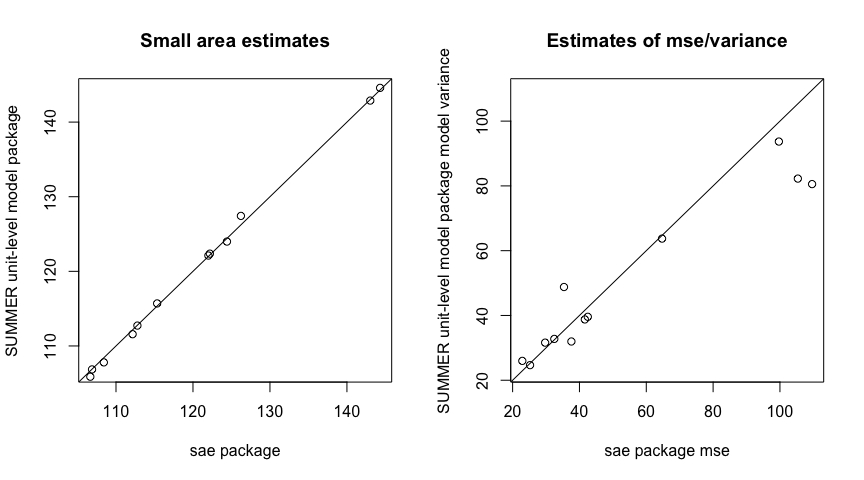### BRFSS Data

Below, we provide an example comparing spatial models from sae and SUMMER using data from the Behavioral Risk Factor Surveillance System (BRFSS).

library(ggplot2)
library(patchwork)
data(BRFSS)
data(KingCounty)
BRFSS <- subset(BRFSS, !is.na(BRFSS$diab2)) BRFSS <- subset(BRFSS, !is.na(BRFSS$hracode))
mat <- getAmat(KingCounty, KingCounty$HRA2010v2_) design <- svydesign(ids = ~1, weights = ~rwt_llcp, strata = ~strata, data = BRFSS) direct <- svyby(~diab2, ~hracode, design, svymean) #### Results using sae Below, we use sae to smooth the logit-transformed direct estimates. direct$var <- direct$se ^ 2 direct$logit.diab2 <- SUMMER::logit(direct$diab2) direct$logit.var <- direct$var / (direct$diab2 ^ 2 * (1 - direct$diab2) ^ 2) SFH.brfss <- sae::mseSFH(logit.diab2 ~ 1, logit.var, mat, data = direct) results <- data.frame(region = direct$hracode,
eblup.SFH = SUMMER::expit(SFH.brfss$est$eblup),
mse = SFH.brfss$mse) #### Results using SUMMER Below, we fit two versions of the spatial area levelmodel in SUMMER. If we change pc.u and pc.alpha from the default value $$u=1,\alpha=0.01$$ to $$u=0.1,\alpha=0.01$$, we assign more prior mass on smaller variance of the random effects, inducing more smoothing. summer.brfss <- smoothArea(diab2~1, domain= ~hracode, design = design, responseType = "binary", Amat = mat, CI = 0.95) summer.brfss.alt <- smoothArea(diab2~1, domain= ~hracode, design = design, responseType = "binary", Amat = mat, CI = 0.95, pc.u = 0.1, pc.alpha = 0.01) Finally, we use SUMMER::mapPlot to compare median estimates and uncertainty estimates obtained via sae and SUMMER. toplot <- summer.brfss$s.dir.sp.est
toplot$logit.var <- toplot$var /
(summer.brfss$s.dir.sp.est$median ^ 2 *
(1 - summer.brfss$s.dir.sp.est$median) ^ 2)
toplot$median.alt <- summer.brfss.alt$s.dir.sp.est$median toplot$logit.var.alt <-  summer.brfss.alt$s.dir.sp.est$var /
(summer.brfss.alt$s.dir.sp.est$median ^ 2 *
(1 - summer.brfss.alt$s.dir.sp.est$median) ^ 2)
toplot$median.sae <- results$eblup.SFH
toplot$mse.sae <- results$mse
variables <- c("median", "median.alt",  "median.sae",
"logit.var", "logit.var.alt", "mse.sae")
names <- c("Median (default prior)", "Median (new prior)",  "EBLUP (sae)",
"Variance (default prior)", "Variance (new prior)", "MSE (sae)")
g1 <- mapPlot(data = toplot, geo = KingCounty,
variables=variables[1:3],
labels = names[1:3], by.data = "domain",
by.geo = "HRA2010v2_", size = 0.1)
g2 <- mapPlot(data = toplot, geo = KingCounty,
variables=variables[4:6], labels = names[4:6],
by.data = "domain", by.geo = "HRA2010v2_", size = 0.1)
g1 / g2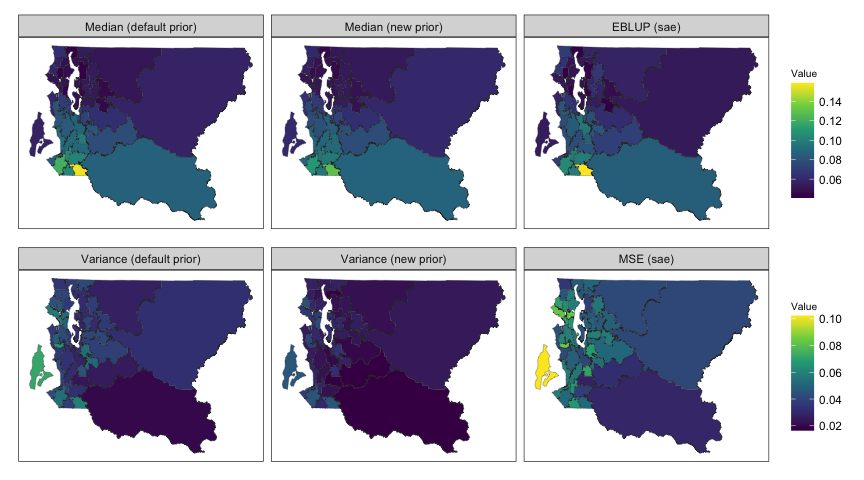par(mfrow = c(1, 2))
range1 <- range(c(direct$diab2,toplot$median.alt))
plot(direct$diab2,toplot$median,
xlab = "direct estimates",
ylab = "model-based estimates",
main = "Small area estimates", col = 'red', pch = 16,
xlim=range1,ylim=range1)
points(direct$diab2,toplot$median.sae,  col = 'blue', pch = 16)
points(direct$diab2,toplot$median.alt,  col = 'cyan', pch = 16)
legend('topleft', pch = 16, col = c('red', 'cyan', 'blue'),
legend = c("SUMMER",'SUMMER (new prior)', "sae"),bty="n")
abline(0,1)
range2 <- range(c(direct$logit.var,toplot$mse.sae,toplot$logit.var.alt)) plot(direct$logit.var,toplot$logit.var, xlab = "direct estimate var.", ylab = "model-based uncertainty", main = "Small area estimates", col = 'red', pch = 16, xlim=range2,ylim=range2) points(direct$logit.var,toplot$mse.sae, col = 'blue', pch = 16) points(direct$logit.var,toplot\$logit.var.alt,  col = 'cyan', pch = 16)
legend('topleft', pch = 16, col = c('red', 'cyan','blue'),
legend = c("SUMMER var.", 'SUMMER var. (new prior)',"sae mse"),bty="n")
abline(0,1)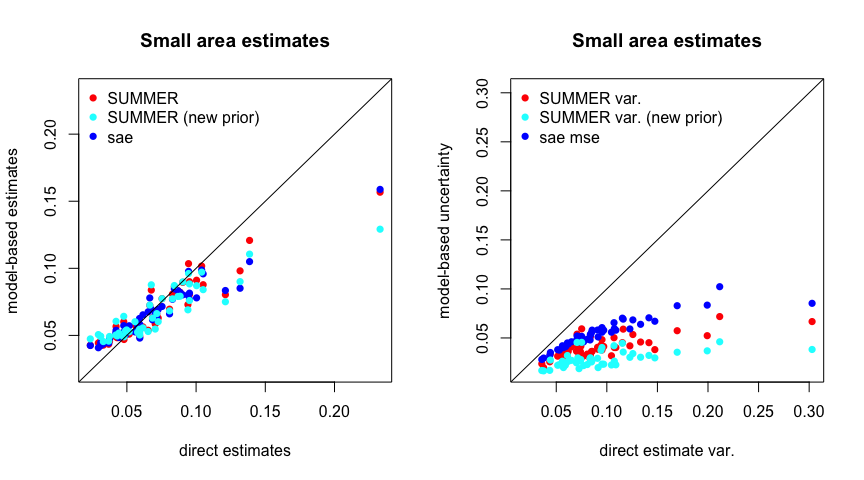Arora, Vipin, and P. Lahiri. 1997. “On the SUPERIORITY OF THE BAYESIAN METHOD OVER THE BLUP IN SMALL AREA ESTIMATION PROBLEMS.” Statistica Sinica 7: 1053–63.
Battese, George E, Rachel M Harter, and Wayne A Fuller. 1988. “An Error-Components Model for Prediction of County Crop Areas Using Survey and Satellite Data.” Journal of the American Statistical Association 83: 28–36.
Fay, R. E., and R. A. Herriot. 1979. “Estimates of Income for Small Places: An Application of James–Stein Procedure to Census Data.” Journal of the American Statistical Association 74: 269–77.
Molina, Isabel, and Yolanda Marhuenda. 2015. “Sae: An R Package for Small Area Estimation.” The R Journal 7: 81–98.
You, Yong, and Beatrice Chapman. 2006. “Small Area Estimation Using Area Level Models and Estimated Sampling Variances.” Survey Methodology 32 (1): 97–103.